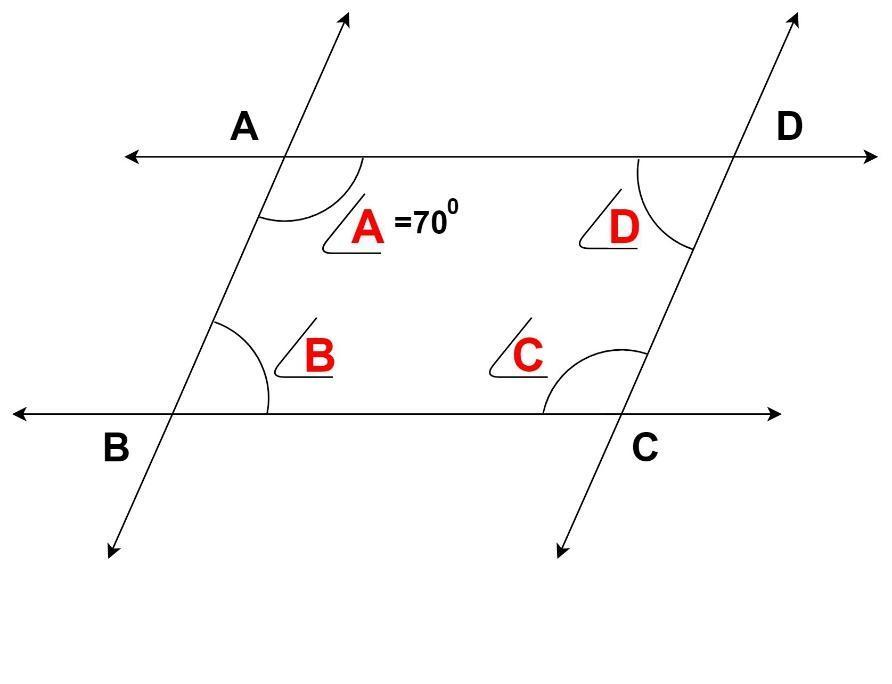Courses
Courses for Kids
Free study material
Free LIVE classes
MoreLIVE
Join Vedantu’s FREE Mastercalss

# ABCD is a parallelogram in which angle $\angle A = {70^0}$ . Compute angle B,angle C and angle D.Verified
363.9k+ views
Hint: Use geometrical theorems related to parallel lines.
Given in the problem ABCD is a parallelogram with angle $\angle A = {70^0}$.
We know that opposite sides of the parallelogram are parallel to each other.
$\Rightarrow AB{\text{ is parallel to }}CD{\text{ (1)}} \\ \Rightarrow AD{\text{ is parallel to B}}C{\text{ (2)}} \\$
Same side Interior angle theorem states that the interior angles formed by a transversal line intersecting two parallel lines are supplementary.
Using the same theorem in parallelogram $ABCD$, we get:
$(1) \Rightarrow {\text{ }}\angle A + \angle D = {180^0}{\text{ and }}\angle B + \angle C = {180^0} \\ (2) \Rightarrow {\text{ }}\angle A + \angle B = {180^0}{\text{ and }}\angle C + \angle D = {180^0} \\$
Using angle $\angle A = {70^0}$ in above equations, we get:
${70^0} + \angle D = {180^0}{\text{ }} \\ \Rightarrow \angle D = {110^0} \\ {70^0} + \angle B = {180^0} \\ \Rightarrow \angle B = {110^0} \\ \angle B + \angle C = {180^0} \\ \Rightarrow \angle C = {70^0} \\$
Hence${\text{ }}\angle B = {110^0}{\text{ , }}\angle C = {70^0}{\text{ , }}\angle D = {110^0}$ .

Note: The same side interior angle theorem is only valid for parallel lines. Geometric properties of parallelogram and parallel lines should be kept in mind while solving problems like this.
Last updated date: 20th Sep 2023
Total views: 363.9k
Views today: 6.63k